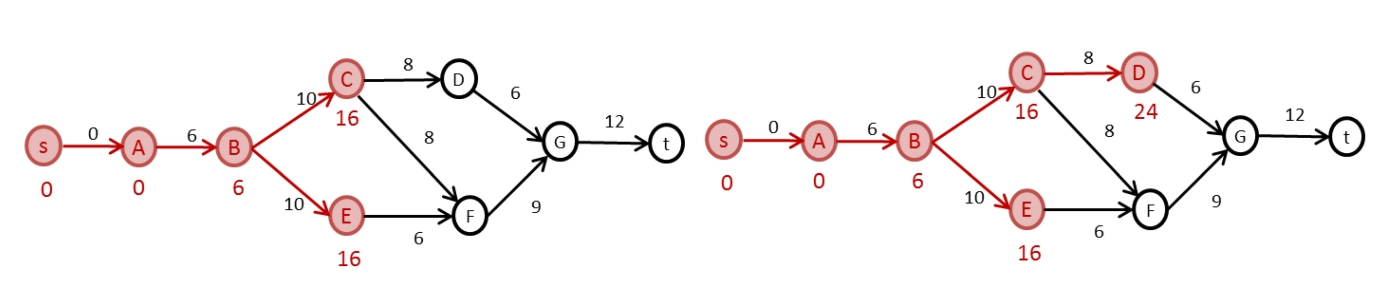# Operations Research

### The Operations Research (OR) Group conducts research and teaching in the theory and applications of OR.

The group's foremost interest is in fundamental research in OR and related areas of mathematics and theoretical computer science, including:
- Methods for solving linear and integer programmes and network flows
- Questions in network reliability
- Average-case analysis of algorithms for graph problems
- Formula satisfiability, and constraint satisfaction problems
- Phase transitions in the same contexts
- Exact algorithms for NP-complete problems

Applications, at levels from commercial development to theoretical research motivated by practical problems, include car and truck fleet scheduling, mobile network efficiency, search games, manufacturing optimisation, computer virus detection and recovery, and DNA sequencing.

The group publish in top-tier OR journals including Mathematical Programming; Mathematics of Operations Research; and Operations Research,and in mathematics and algorithms journals including Journal of Computational Theory (Series B); ACM Transactions on Algorithms; Combinatorica; Combinatorics, Probability and Computing;and SIAM Journal on Computing, as well as in conferences including FOCS, STOC, and SODA.

The group enjoys close ties with the LSE's Department of Management.

Individual faculty interests are listed below.

## A

• Packing and covering
• Ideal clutters
• Perfect graphs
• Integral polyhedra
• Discrete geometry

## O

Dr Neil Olver

• Combinatorial optimisation and its intersections with algorithmic game theory and probability
• Designing provably good algorithms for fundamental network optimisation problems, motivated by applications such as road traffic and telecommunication networks
• Network design

## P

• Patrolling games on networks for security of infrastructures
• Approximate dynamic programming algorithms: transportation, wireless telecommunication, financial portfolio optimization
• Robust optimization: efficient operation of wireless networks
• Resource management on wireless networks: combinatorial optimisation algorithms for Scheduling, Routing and Energy Efficiency
• Game theory on networks: cooperative and non-cooperative

## S

Professor Gregory Sorkin

• Combinatorial optimization
• Phase transitions in random structures including random graphs and formulae
• Average-case analysis of algorithms
• Exponential-time algorithms
• Graph theory, combinatorics, and the probabilistic method
• Applications in operations research, biology, physics, auctions, and other areas

## V

Dr László Végh

• Combinatorial optimisation
• Graph theory
• Discrete mathematics
• Algorithmic game theory

Dr Victor Verdugo

• Approximation Algorithms
• Online Optimisation
• Algorithmic Game Theory
• Estimation and Dynamics in Networks

## Z

Dr Giacomo Zambelli

• Integer programming
• Combinatorial optimization
• Polyhedral combinatorics
• 0/1 matrices
• Graph theory